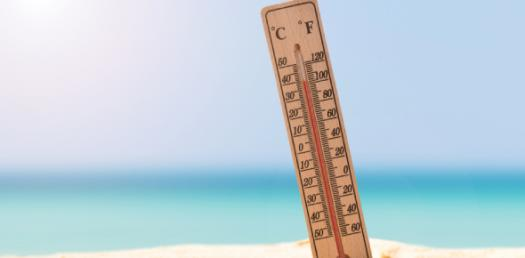# What Do You Know About Heat And Temperature? Trivia Quiz

23 Questions | Total Attempts: 113Settings.

• 1.
Define "temperature".
• A.

Temperature is how hot or cold an object is.

• B.

Temperature is how you feel when you touch an object.

• C.

Temperature is hotness or coldness of an object.

• D.

Temperature is a measurement of the degree of "hotness" of an object.

• 2.
Is your sense of touch good enough for measuring temperature?
• A.

Yes.

• B.

No.

• 3.
What is the units for temperature?
• A.

Metres.

• B.

Centimetre-cube.

• C.

Degree Celsius.

• D.

Degree.

• 4.
What happens to the temperature and the liquid level of the thermometer when it is placed in cold water?
• A.

The temperature drops and the liquid level rises.

• B.

The temperature rises and the liquid level rises.

• C.

The temperature drops and the liquid level rises.

• D.

The temperature drops and the liquid level drops.

• 5.
Rising warm air currents is an example of
• A.

• B.

Conduction

• C.

Convection

• 6.
A thermometer works because the liquid in it contracts when heated this is an example of
• A.

• B.

Conduction

• C.

Convection

• 7.
Higher temperature means faster-moving molecules.
• A.

True

• B.

False

• 8.
Aair is a great conductor of heat.
• A.

True

• B.

False

• 9.
The reason why water boils causing the circular motion is due to?
• A.

• B.

Conduction

• C.

Convection

• 10.
When you get into a car with hot black leather in the middle of the summer and you get your buns toasted, this is an example of?
• A.

• B.

Conduction

• C.

Convection

• 11.
When rocks are heated by the warmth of the sun this is an example of?
• A.

• B.

Conduction

• C.

Convection

• 12.
When a metal spoon with a temperature of 20oC is placed into a cup of water with a temperature of 90oC the spoon will heat up. This is an example of:
• A.

• B.

Conduction

• C.

Convection

• 13.
During a house fire, the smoke and flames rise up, but the air down near the floor is cooler and less smoky. This is an example of
• A.

• B.

Conduction

• C.

Convection

• 14.
When heat is given off by light this type of heat is known as?
• A.

• B.

Conduction

• C.

Convection

• 15.
The transfer of heat between substances that are in direct contact with each other is called what?
• A.

• B.

Convection

• C.

Conduction

• D.

Heat Transfer

• 16.
The rays in this picture is an example of
• A.

Conduction

• B.

Convection

• C.

• 17.
The air in the hot air balloon is heated and circulates.  This is an example of ...
• A.

Conduction

• B.

Convection

• C.

• 18.
You walk barefoot on the hot street and it burns your toes.  This is an example of...
• A.

Conduction

• B.

Convection

• C.

• 19.
A boy sits to the side of a campfire.  He is 10 feet away, but still feels warm.  This is an example of ...
• A.

Conduction

• B.

Convection

• C.

• 20.
Light waves traveling through empty space.
• A.

Conduction

• B.

Convection

• C.

• 21.
Absolute Zero is known as...?
• A.

The temperature that water freezes

• B.

The temperature where particles of matter vibrate more

• C.

The temperature where particles of matter vibrate almost to a stop

• D.

The temperature where water boils

• 22.
What is thermal expansion?
• A.

The increase in volume of a substance because of an increase in temperature

• B.

The decrease in volume of a substance because of a decrease in temperature

• C.

The increase in volume of a substance because of a decrease in temperature

• D.

The decrease in space an object takes up because of an increase in temperature

• 23.
How is air heated in our atmosphere?
• A.Back to top